# Search

About 14 Search Results Matching Types of Worksheet, Worksheet Section, Generator, Generator Section, Subjects matching Geometry, Type matching Worksheet, Similar to Easy Measurement Worksheets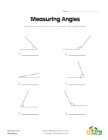## Measuring Angles Worksheet 1

Measure each of the angles with a protractor and w...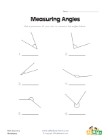## Measuring Angles Worksheet 2

Use a protractor to measure each of the angles.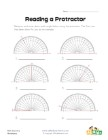## Reading a Protractor Worksheet 2

Read each protractor and write down the angles the...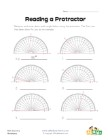## Reading a Protractor Worksheet 1

Read each protractor and write down the angles the...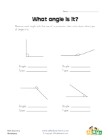## What Angle Is It?

Measure each angle and decide what type of angle i...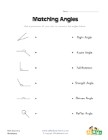## Matching Angles Worksheet

Draw a line to match each of the angles with the t...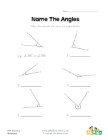## Name the Angles Worksheet

Follow the example and name each of the angles.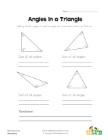## Angles in a Triangle Worksheet

Kids learn that the angles of a triangle always ad...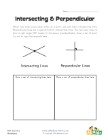## Intersecting and Perpendicular Lines Worksheet

Learn about intersecting and perpendicular lines.